Quantitative MCQ - 17

# Quantitative MCQ - 17

Test Description

## 30 Questions MCQ Test Quantitative Aptitude for Competitive Examinations | Quantitative MCQ - 17

Quantitative MCQ - 17 for Quant 2022 is part of Quantitative Aptitude for Competitive Examinations preparation. The Quantitative MCQ - 17 questions and answers have been prepared according to the Quant exam syllabus.The Quantitative MCQ - 17 MCQs are made for Quant 2022 Exam. Find important definitions, questions, notes, meanings, examples, exercises, MCQs and online tests for Quantitative MCQ - 17 below.
Solutions of Quantitative MCQ - 17 questions in English are available as part of our Quantitative Aptitude for Competitive Examinations for Quant & Quantitative MCQ - 17 solutions in Hindi for Quantitative Aptitude for Competitive Examinations course. Download more important topics, notes, lectures and mock test series for Quant Exam by signing up for free. Attempt Quantitative MCQ - 17 | 30 questions in 30 minutes | Mock test for Quant preparation | Free important questions MCQ to study Quantitative Aptitude for Competitive Examinations for Quant Exam | Download free PDF with solutions
 1 Crore+ students have signed up on EduRev. Have you?
Quantitative MCQ - 17 - Question 1

### Directions (1-5): What approximate value should come in place of the question mark (?) in the following questions? (10.97)² + (4.03)³ × 3.99 = ?

Detailed Solution for Quantitative MCQ - 17 - Question 1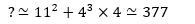Quantitative MCQ - 17 - Question 2

### Directions (1-5): What approximate value should come in place of the question mark (?) in the following questions? 12.10% of 935.01 + 1499.98% of 25.95 = ?

Detailed Solution for Quantitative MCQ - 17 - Question 2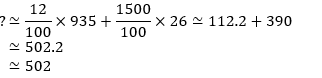Quantitative MCQ - 17 - Question 3

### Directions (1-5): What approximate value should come in place of the question mark (?) in the following questions? ∛65×23.93-31.04= ?

Detailed Solution for Quantitative MCQ - 17 - Question 3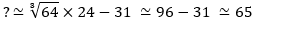Quantitative MCQ - 17 - Question 4

Directions (1-5): What approximate value should come in place of the question mark (?) in the following questions?

1624.02 × 3.991 = ?

Detailed Solution for Quantitative MCQ - 17 - Question 4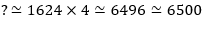Quantitative MCQ - 17 - Question 5

Directions (1-5): What approximate value should come in place of the question mark (?) in the following questions?

3018.01 ÷ 2.97 – 841.02 = ?

Detailed Solution for Quantitative MCQ - 17 - Question 5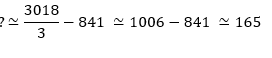Quantitative MCQ - 17 - Question 6

Two vessels contain the spirit of 0.5 and 0.75 concentrations. If 2 litres from the first vessel and 3 litres from the second vessel are mixed, then what will be the ratio of the spirit and the water in the resultant solution?

Detailed Solution for Quantitative MCQ - 17 - Question 6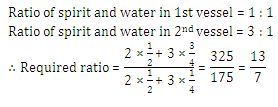Quantitative MCQ - 17 - Question 7

In a mixture of 60 litres, the ratio of milk and water is 2 : 1. If the ratio of the milk and water is to be 1 : 2, then the amount of water to be further added must be

Detailed Solution for Quantitative MCQ - 17 - Question 7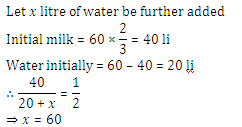Quantitative MCQ - 17 - Question 8

A person has a chemical of Rs. 25 per litre. In what ratio should water be mixed in that chemical so that after selling the mixture at Rs. 20 per litre he may get a profit of 25%?

Detailed Solution for Quantitative MCQ - 17 - Question 8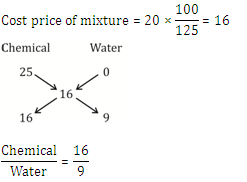Quantitative MCQ - 17 - Question 9

How many kg of sugar costing Rs. 5.75 per kg should be mixed with 75 kg of cheaper sugar costing Rs. 4.50 per kg so that the mixture is worth Rs. 5.50 kg?

Detailed Solution for Quantitative MCQ - 17 - Question 9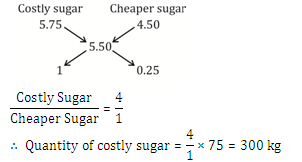Quantitative MCQ - 17 - Question 10

In a zumba class, the average age of all the members was 43.5 years. 10 members left the class and 6 new members joined. If the average age increased by 2 years and the total age decreased by 110, what was the number of members in the class initially?

Detailed Solution for Quantitative MCQ - 17 - Question 10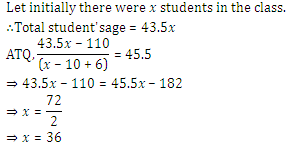Quantitative MCQ - 17 - Question 11

There are 5 consecutive odd numbers. If the difference between the square of the average of first two odd numbers and the square of the average of last two odd numbers is 588, what is the smallest odd numbers?

Detailed Solution for Quantitative MCQ - 17 - Question 11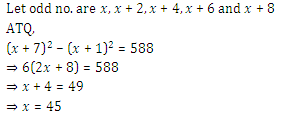Quantitative MCQ - 17 - Question 12

Directions (Q12,13): What should come in place of question mark (?) in following simplification problems?

0.009 + 0.001 ÷ 10 + 0.003 = ?

Detailed Solution for Quantitative MCQ - 17 - Question 12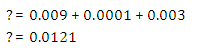Quantitative MCQ - 17 - Question 13

Directions (Q12,13): What should come in place of question mark (?) in following simplification problems?

48% of 950 – 46% of 840 = ?

Detailed Solution for Quantitative MCQ - 17 - Question 13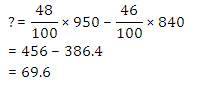Quantitative MCQ - 17 - Question 14

Directions (14-18): Study the pie-charts carefully to answer the questions that follow:

Percentage of employees and women working in different Departments of an Organisation

Percentage of employees working in different department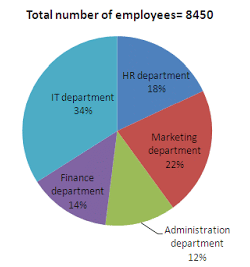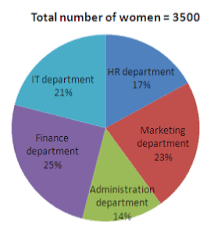Which department has the lowest number of Men working in it?

Detailed Solution for Quantitative MCQ - 17 - Question 14

From pie chart we can say that two different departments i.e. administration and finance have number of men employee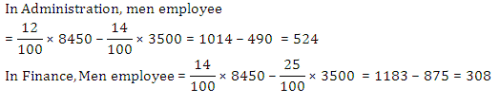Quantitative MCQ - 17 - Question 15

Directions (14-18): Study the pie-charts carefully to answer the questions that follow:

Percentage of employees and women working in different Departments of an Organisation

Percentage of employees working in different departmentWhat is the total number of men working in HR, Marketing and IT departments together in the organisation?

Detailed Solution for Quantitative MCQ - 17 - Question 15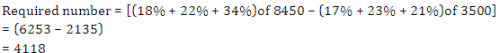Quantitative MCQ - 17 - Question 16

Directions (14-18): Study the pie-charts carefully to answer the questions that follow:

Percentage of employees and women working in different Departments of an Organisation

Percentage of employees working in different departmentWhich is the respective ratio of the number of women working in Administration department to the total number of employees working in that department?

Detailed Solution for Quantitative MCQ - 17 - Question 16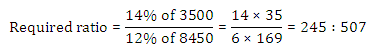Quantitative MCQ - 17 - Question 17

Directions (14-18): Study the pie-charts carefully to answer the questions that follow:

Percentage of employees and women working in different Departments of an Organisation

Percentage of employees working in different departmentThe number of women working in the Finance and IT department together form what percent of total number of employees in the organisation? (rounded off to two digits after decimal)

Detailed Solution for Quantitative MCQ - 17 - Question 17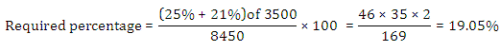Quantitative MCQ - 17 - Question 18

Directions (14-18): Study the pie-charts carefully to answer the questions that follow:

Percentage of employees and women working in different Departments of an Organisation

Percentage of employees working in different departmentWhat is the number of men working in the HR department?

Detailed Solution for Quantitative MCQ - 17 - Question 18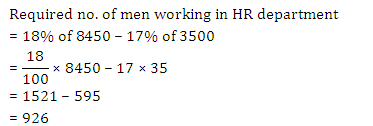Quantitative MCQ - 17 - Question 19

The wheat sold by a grocer contained 10% low quality wheat. What quantity of good quantity wheat should be added to 150 kg of wheat so that the percentage of low-quality wheat becomes 5%?

Detailed Solution for Quantitative MCQ - 17 - Question 19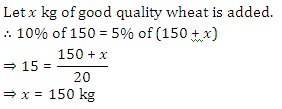Quantitative MCQ - 17 - Question 20

Two pipes can fill a cistern in 14 and 16 hours respectively. The pipes are opened simultaneously and it is found that due to leakage in the bottom of the cistern, it takes 32 minutes extra for the cistern to be filled up. When the cistern is full, in what time will the leak empty it?

Detailed Solution for Quantitative MCQ - 17 - Question 20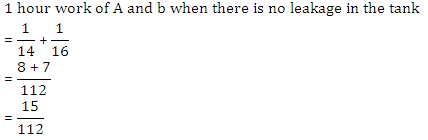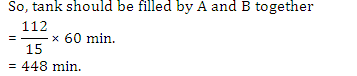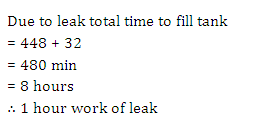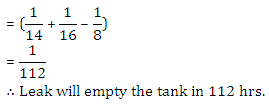Quantitative MCQ - 17 - Question 21

The ratio between the speed of a train and a car is 18 : 3 respectively. Also, a bus covered a distance of 480 kms in 12 hours. The speed of the bus is five-ninths the speed of the train. How much distance will the car cover in 5 hours?

Detailed Solution for Quantitative MCQ - 17 - Question 21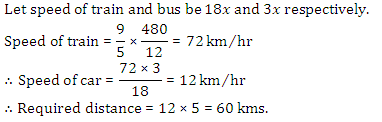Quantitative MCQ - 17 - Question 22

Train-A crosses a pole in 25 seconds and another Train-B crosses as pole in 1 minute and 15 seconds. The Length of the train-A is half the length of the train-B. What is the respective ratio between the speed of the train-A and the train-B?

Detailed Solution for Quantitative MCQ - 17 - Question 22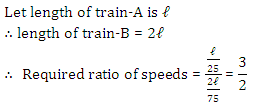Quantitative MCQ - 17 - Question 23

Directions (23-25): What value should come in the place of question mark (?) in the following questions?

3.6 + 36.6 + 3.66 + 0.36 + 3.0 = ?

Detailed Solution for Quantitative MCQ - 17 - Question 23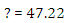Quantitative MCQ - 17 - Question 24

Directions (23-25): What value should come in the place of question mark (?) in the following questions?

23 × 45 ÷ 15 = ?

Detailed Solution for Quantitative MCQ - 17 - Question 24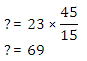Quantitative MCQ - 17 - Question 25

Directions (23-25): What value should come in the place of question mark (?) in the following questions?

83% of 2350 = ?

Detailed Solution for Quantitative MCQ - 17 - Question 25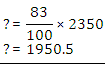Quantitative MCQ - 17 - Question 26

Directions (6-10): In the given question, two equations numbered I and II are given. You have to solve both the equations and Give answer:

I. 2x² - 7x+ 3 = 0

II. 2y² - 7y + 6 = 0

Detailed Solution for Quantitative MCQ - 17 - Question 26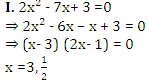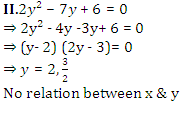Quantitative MCQ - 17 - Question 27

Directions (6-10): In the given question, two equations numbered I and II are given. You have to solve both the equations and Give answer:

I. 4x² + 16x +15 = 0

II. 2y² + 3y + 1 = 0

Detailed Solution for Quantitative MCQ - 17 - Question 27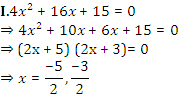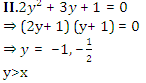Quantitative MCQ - 17 - Question 28

Directions (6-10): In the given question, two equations numbered I and II are given. You have to solve both the equations and Give answer:

I. 9x² - 45x + 56 = 0

II. 4y² - 17y + 18 = 0

Detailed Solution for Quantitative MCQ - 17 - Question 28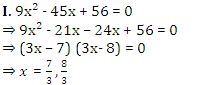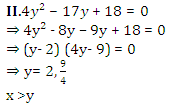Quantitative MCQ - 17 - Question 29

Directions (6-10): In the given question, two equations numbered I and II are given. You have to solve both the equations and Give answer:

I. 2x² + 11x + 14 = 0

II. 2y² + 15y + 28 = 0

Detailed Solution for Quantitative MCQ - 17 - Question 29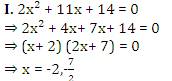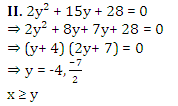Quantitative MCQ - 17 - Question 30

Directions (6-10): In the given question, two equations numbered I and II are given. You have to solve both the equations and Give answer:

I. 6x² + 11x + 4 = 0

II. 4y² - 7y – 2 = 0

Detailed Solution for Quantitative MCQ - 17 - Question 30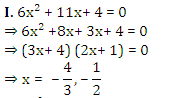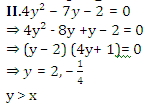## Quantitative Aptitude for Competitive Examinations

33 videos|34 docs|143 tests
 Use Code STAYHOME200 and get INR 200 additional OFF Use Coupon Code
Information about Quantitative MCQ - 17 Page
In this test you can find the Exam questions for Quantitative MCQ - 17 solved & explained in the simplest way possible. Besides giving Questions and answers for Quantitative MCQ - 17, EduRev gives you an ample number of Online tests for practice

## Quantitative Aptitude for Competitive Examinations

33 videos|34 docs|143 tests